### Python Lab Tutorials: Lab 11

Consider the following BVP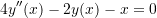With the boundary conditions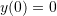and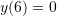• Find the exact solution using Sympy’s built-in “dsolve” function.
• Use the finite difference method with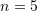intervals to find a numerical solution.
• Plot the exact solution overlapping the data points obtained using the numerical solution.
• Find the maximum absolute error.
• Write a procedure whose input isand the output is the maximum absolute error.
• Plot the curve of the maximum absolute error vs.for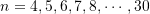• Plot the curve of the maximum absolute error vs.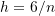for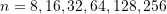• Fit the nonlinear model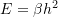to the data of maximum error vs. h (Note the error is expected to be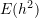because the basic formula for the centered finite difference scheme is used)